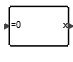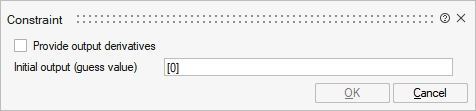# Constraint

This block outputs values so that its input remains zero. This works only if there is a path back from its output to its input in the diagram. If F is the function corresponding to this path, the block solves the equation F(x)=0 where x denotes the output of the block. If the solver cannot find an initial solution, you can provide an initial guess value for the output. If F(x)=0 has several solutions, the initial guess helps the solver to select the desired solution. It is also possible to make use of the output derivative to build the F function, such as F(xapos;,x)=0. In this case, the guess values for x and its derivative xapos; should be provided.## Library

Activate/MathOperations

## Description

The Algebraic Constraint block constrains the input signal f(x) to zero and outputs an algebraic state x. The block outputs the value necessary to produce a zero at the input.

The output must affect the input through some direct feedback path, i.e., the feedback path should only contain blocks with direct feedthrough (without internal states). This enables you to specify algebraic equations for index-1 differential/algebraic systems (DAEs). By default, the initial value of the state which is used as initial guess values is zero.

If the solver cannot converge towards the solution at the beginning of the integration, you can help the solver by providing an initial guess that is close to the solution value. If f(x) has several solutions, the initial guess helps the solver to select the desired solution.

## ParametersNameLabelDescriptionData TypeValid Values

dae

Provide output derivatives

Number

0
1

x0

Initial output (guess value)

real value. default value of the state is 0.

Matrix

xdf

initial output derivative (guess value)

Structure

xdf/xd

Initial state derivative

Cell of matrices

## Ports

NameTypeDescriptionIO TypeNumber

x

explicit

output

1

Port 2

explicit

output

dae

=0

explicit

input

1

NameValueDescription

always active

yes

direct-feedthrough

no

zero-crossing

no

mode

no

continuous-time state

yes

discrete-time state

no